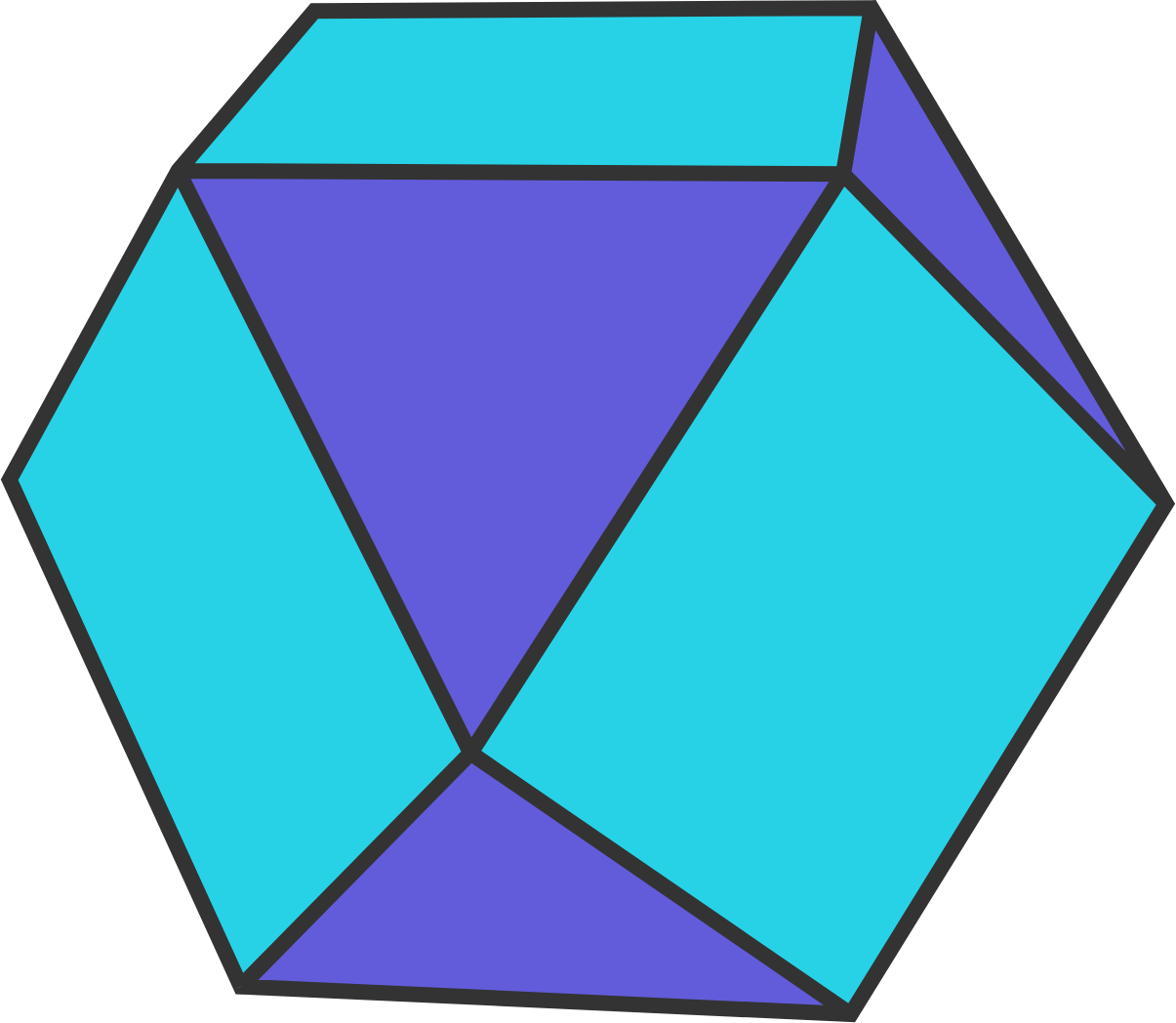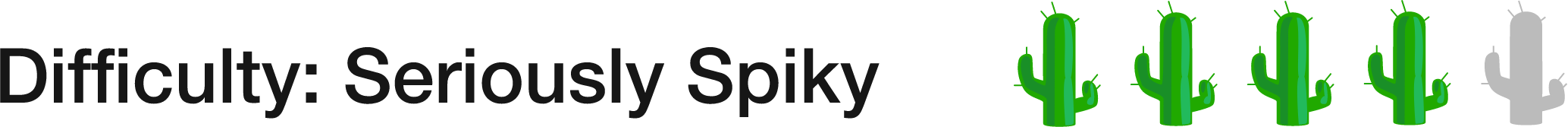# 65 of 100: It's Also Called A Heptaparallelohedron

Geometry Level 1A cuboctahedron is a three dimensional polyhedron where at each vertex there are two squares and two equilateral triangles arranged as shown. Which of these numbers is greater?

A. The number of triangular faces

B. The number of square facesFocus on the relationship between the faces (that is, the the triangles and squares) and the vertices (where four of them meet).

×